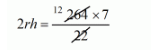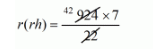# The curved surface area of a cylinder is 264 m2`
Question:

The curved surface area of a cylinder is 264 m2 and its volume is 924 m3. The ratio of its diameter to its height is

(a) 3 : 7

(b) 7 : 3

(c) 6 : 7

(d) 7 : 6

Solution:

The C.S.A. of cylinder

S = 264 m2

The volume of cylinder

V = 924 m3

$2 \pi r h=264$....................(i)

$2 r h=84$

$r h=42$

$\pi r^{2} h=924$...........$. .(i i)$

$r(r h)=42 \times 7$

From eq. (i) and (ii),

We get $r=7$

Putting the value in $(i) \quad h=6$

Hence, $\frac{d}{h}=\frac{14}{6}=\frac{7}{3}$

$d: h=7: 3$

Hence, the correct answer is choice (b).